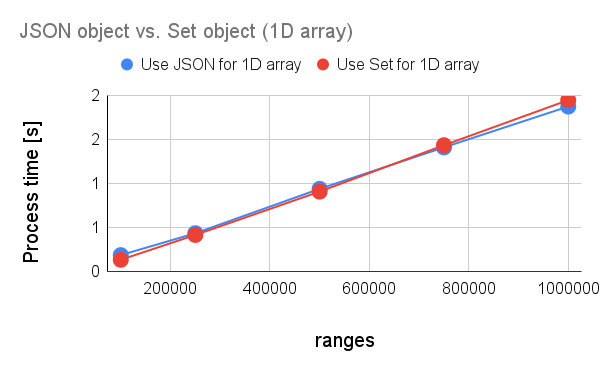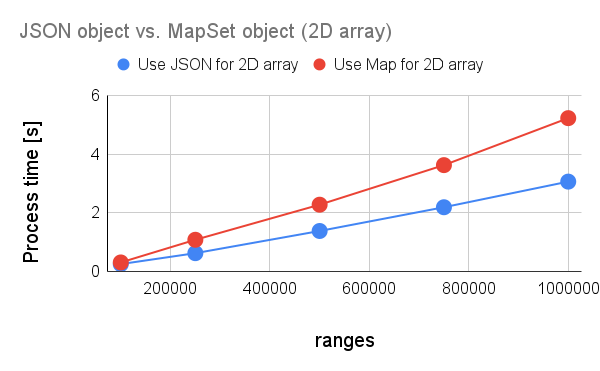# Benchmark: Process Costs for Searching Value using Object with Google Apps Script

Gists

When a value is searched from the 1-dimensional array and a 2-dimensional array, after V8 runtime could be used, I use JSON object, Set object, and Map Object. But, I had never measured the process cost of this situation. In this post, I would like to introduce the process cost for searching a value using a JSON object, Set object, and Map object converted from the 1-dimensional array and 2-dimensional array.

## Experimental procedure

In this report, I used 1 dimensional array and 2 dimensional array as the sample input arrays. You can see them in “Appendix” section.

In order to measure the process cost, it was measured by changing the number of element in the array. About 2 dimensional array, in this case, the length of each element is the same. The number of element is changed. You can see the scripts for searching values in “Appendix” section.

By the way, at GAS, the processing time is not stable as you know. So in this report, the average value for more than 600 times measurements was used for each data point which is shown by the figures. At this time, the fluctuation of the average values was less than 1 %. I worry that each detailed-data point in my environment might be different from that in another user’s environment. But I think that the trend of this result can be used.

## Results and discussionsFig1. Process cost for searching a value using JSON object and Set object from the 1-dimensional array.

From Fig. 1, it was found that the process costs for searching a value using JSON object and Set object from the 1-dimensional array are almost the same.Fig2. Process cost for searching a value using JSON object and Map object from the 2-dimensional array.

From Fig. 2, it was found that in the case of the process costs for searching a value using JSON object and Map object from a 2-dimensional array, the process cost for searching using JSON object is about 50 % lower than that for searching using Map object. But, both process cost is much lower than that of the method without using the objects.

When the methods for using JSON object and Map object are used, there are the following important points.

When JSON object is used, the order of properties is not preserved. When the Map object is used, the order of properties is preserved.

## Appendix

### Sample arrays

#### 1 dimensional array

const ar = ["key0000000001","key0000000002","key0000000003",,,,]


#### 2 dimensional array

const ar = [
["key0000000001","valueA_0000000001","valueB_0000000001","valueC_0000000001","valueD_0000000001","valueE_0000000001"],
["key0000000002","valueA_0000000002","valueB_0000000002","valueC_0000000002","valueD_0000000002","valueE_0000000002"],
["key0000000003","valueA_0000000003","valueB_0000000003","valueC_0000000003","valueD_0000000003","valueE_0000000003"],
,
,
,
]


### Scripts

// For 1D Using JSON object
const obj = Object.fromEntries(ar.map((k) => [k, true]));
const res = ar.every((a) => a in obj);

// For 1D Using Set object
const obj = new Set(ar);
const res = ar.every((a) => obj.has(a));

// For 2D Using JSON object
const obj = Object.fromEntries(ar.map(([k, ...v]) => [k, v]));
const res = ar.every(([a]) => a in obj);

// For 2D Using Map object
const obj = new Map(ar.map(([k, ...v]) => [k, v]));
const res = ar.every(([a]) => obj.has(a));